# Combining repulsion and nudging

## Introduction

The very popular R package ‘ggrepel’ does a great job at avoiding overlaps among data labels and between them and observations plotted as points. A difficulty that stems from the use of an algorithm based on random displacements is that the final location of the data labels can become more disordered than necessary. In addition when including smooth regression lines the data labels may partly occlude the fitted line and/or the confidence band.

Package ‘ggpp’ defines new position functions that save the starting position like position_nudge_repel() does but come in multiple flavors. Their use together with repulsive geometries from ‘ggrepel’ makes it possible to give to the data labels an initial “push” in a non-random direction. This helps a lot, much more than what I expect initially, in obtaining a more orderly displacement by repulsion of the data labels away from a cloud of observations or a line.

Another problem sometimes encountered when using position functions is that combinations of pairs of displacements would be required. ‘ggpp’ does define such new position functions which can also be used together with the repulsive geometries from package ‘ggrepel’.

Because of the naming convention used, the new position functions remain fully compatible with all geometries that have a formal parameter position. However, most examples below use geometries from packages ‘ggrepel’ or ‘ggpp’ to create a plot layer containing data labels.

## Preliminaries

As we will use text and labels on the plotting area we change the default theme to an uncluttered one.

library(dplyr)
library(lubridate)
library(ggplot2)
library(ggpp)
library(grid)
# Is a compatible version of 'ggrepel' installed?
eval_ggrepel <- requireNamespace("ggrepel", quietly = TRUE) &&
packageVersion("ggrepel") >= "0.9.2"
if (eval_ggrepel) library(ggrepel)

old_theme <- theme_set(theme_bw())

## Position functions and nudging

Nudging shifts deterministically the x and/or y coordinates of an observation. This takes place early enough for the limits of the corresponding scales be set based on the displaced positions. In ‘ggplot2’, position functions and consequently also geometries by default apply no nudging.

Function position_nudge() from package ‘ggplot2’ applies the nudge, to x and/or y data coordinates based directly on the values passed to its parameters x and y. Passing arguments to the nudge_x and/or nudge_y parameters of a geometry has the same effect, as these values are passed to position_nudge() within the geometry’s code. Geometries also have a position parameter to which we can pass an expression based on a position function which opens the door to more elaborate approaches to nudging, as well as allowing other changes in coordinates such as stacking.

We use geom_point_s() to exemplify what nudging does. The black dots are the original positions and the red ones the nudged positions, with the arrows of length 0.5 along x, showing the displacement and its direction.

ggplot(data.frame(x = 1:10, y = rnorm(10)), aes(x, y)) +
geom_point() +
geom_point_s(nudge_x = 0.5, colour = "red")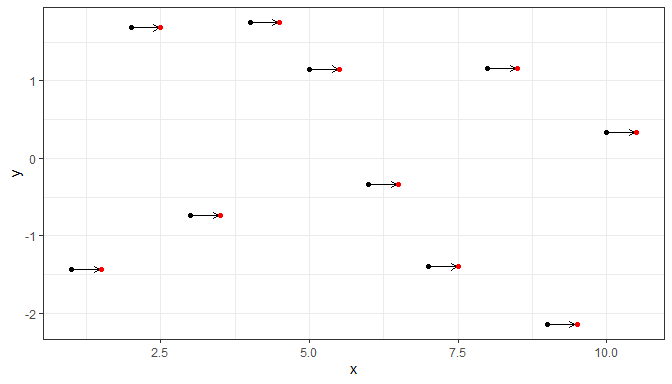Function position_nudge_keep() keeps a copy of the original position making it possible for geometries like geom_point_s() to draw connecting segments or arrows.

Package ‘ggpp’ provides several new position functions to facilitate nudging. All of them keep the original positions to allow links to be drawn. Some of them, just simplify some use cases, e.g., position_nudge_to(), which accepts the desired nudged coordinates directly, instead of as a displacement away from the initial position. This allows to push data labels away from observations into a row or column.

Other new position functions compute the nudge for individual observations based on different criteria. For example by nudging away from a focal point, a line or a curve. The focal point or line can be either supplied directly or fitted to the observations. In position_nudge_center() and position_nudge_line() described below, this reference alters only the direction (angle) along which nudge is applied but not the extent of the shift. Advanced nudging works very well, but only for some patterns of observations and may require manual adjustment of positions, repulsion is more generally applicable but like jittering is aleatory. Combining nudging and repulsion we can make repulsion more predictable with little loss of its applicability.

These position functions can be used with any geometry but if segments joining the nudged positions to the original ones are desired, only geometries from packages ‘ggrepel’ or ‘ggpp’ can currently be used. Geometries geom_text_repel() or geom_label_repel() from ‘ggrepel’ should be used when repulsion is desired. Setting max.iter = 0 in these functions disables repulsion but allows the drawing of segments or arrows. Alternatively, several geometries from ‘ggpp’ implement the drawing of connecting segments, but none of them implement repulsion. Please see the documentation for the different geometries from packages ‘ggrepel’ and ‘ggpp’ for the details.

As mentioned above, drawing of segments or arrows is made possible by position functions storing in data both the nudged and original x and y coordinates. The joint use of ‘ggrepel’ and ‘ggpp’ was made possible by coordinated development of these packages and agreement on a naming convention for storing the original position. Keeping both nudged and original positions increases the size of the data, and consequently also the size of the ggplot objects. Because of this, the position functions from ‘ggpp’ allow the keeping of the original positions to be disabled when needed.

### Connecting segments and arrows

Function position_nudge_keep() is like ggplot2::position_nudge() but keeps (stores) the original x and y coordinates. It is similar to function position_nudge_repel() but uses a different naming convention for the coordinates. Both work with geom_text_repel() or geom_label_repel() from package ‘ggrepel’ (>= 0.9.2), but only position_nudge_keep() can be used interchangeably with ggplot2::position_nudge() with other geometries such as geom_text().

set.seed(84532)
df <- data.frame(
x = rnorm(20),
y = rnorm(20, 2, 2),
l = paste("label:", letters[1:20])
)

With position_nudge_keep() from ‘ggpp’ used together with geom_text_repel() or geom_label_repel() segments between a nudged and/or repelled label and the original position (here indicated by a point) are drawn. As shown here, passing max.iter = 0 disables repulsion.

ggplot(df, aes(x, y, label = l)) +
geom_point() +
geom_text_repel(position = position_nudge_keep(x = 0.3),
max.iter = 0)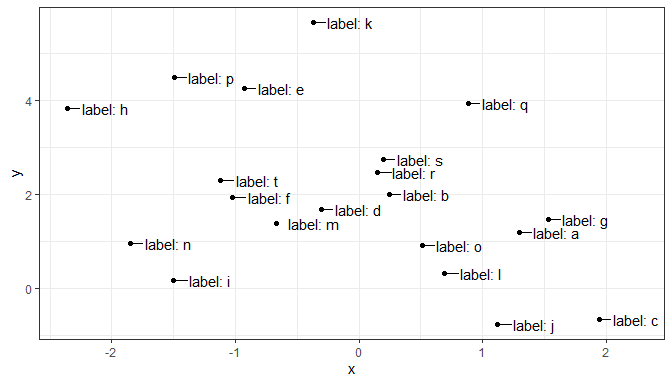With position_nudge() from ‘ggplot2’ used together with geom_text_repel() or geom_label_repel() segments connecting a nudged and/or repelled label and the original position (here indicated by a point) are not drawn.

position_nudge_keep() and all other position functions from ‘ggpp’, described below, can be used with all ‘ggplot2’ geometries but the original position will be ignored and no connecting segment drawn unless the geometry has been designed to work together with them. Currently, geom_text_repel() and geom_label_repel() from ‘ggrepel’ and geom_text_s(), geom_label_s(), geom_point_s(), geom_plot(), geom_table() and geom_grob() from package ‘ggpp’ draw connecting segments.

### Differences among geometries

The geometries from ‘ggrepel’ and ‘ggpp’ can interoperate. However, these geometries are different in several respects. The simpler geometries from ‘ggpp’ add a few features but lack several features compared to geom_text_repel() and geom_label_repel(). First of all, the geometries from ‘ggpp’ do not support repulsion. Those from ‘ggpp’ allow aesthetic mappings to be selectively applied to the different components of the label and/or to segments. However, they do not support aesthetics affecting the segments. While geom_text_repel() and geom_label_repel() support curved connecting segments and arrows, the geometries from ‘ggpp’ support only straight segments and arrows.

Another important difference is that the geometries from the two packages use by default a different approach to justification of the displaced data labels. The geometries from ‘ggpp’ by default justify the text or label to the nearest edge to the original position (thus, away from it). This new justification approach named "position" in ‘ggpp’ is not yet available in geometries defined in ‘ggrepel’.

ggplot(df, aes(x, y, label = l)) +
geom_point() +
geom_text_s(position = position_nudge_keep(x = 0.1),
min.segment.length = 0) +
expand_limits(x = 2.3)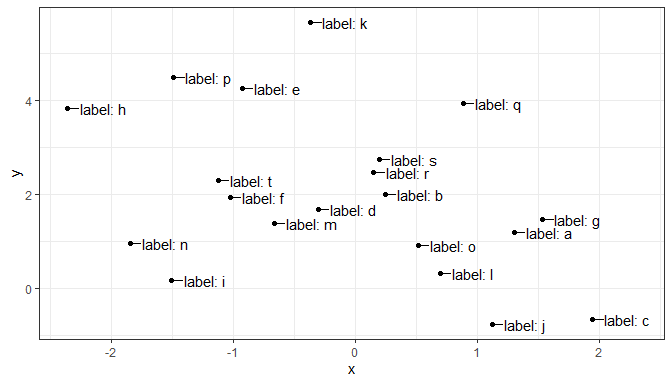geom_text_repel() draws the segment by default from the centre of the text and trims it to the edge of the text plus the padding. In contrast, geom_text_s() uses justification to avoid the overlap and only the default justification "position" and one of the edges, “left” in this case, are currently usable as the untrimmed segment otherwise overplots the text (not shown).

ggplot(df, aes(x, y, label = l)) +
geom_point() +
geom_text_s(position = position_nudge_keep(x = 0.1),
min.segment.length = 0,
hjust = "left") +
expand_limits(x = 2.3)Each approach has advantages and disadvantages. The main difference is that with geom_text_s() and geom_label_s() shorter nudging displacements may be needed than with geom_text_repel() and geom_label_repel() when using their respective default justification approaches.

A usually more problematic example is the labeling of loadings in PCA and similar biplots.

## Example data frame where each species' principal components have been computed.
df1 <- data.frame(
Species = paste("Species",1:5),
PC1     = c(-4, -3.5, 1, 2, 3),
PC2     = c(-1, -1, 0, -0.5, 0.7))

ggplot(df1, aes(x=PC1, y = PC2, label = Species, colour = Species)) +
geom_hline(aes(yintercept = 0), linewidth = .2) +
geom_vline(aes(xintercept = 0), linewidth = .2) +
geom_segment(aes(x = 0, y = 0, xend = PC1, yend = PC2),
arrow = arrow(length = unit(0.1, "inches"))) +
geom_label_repel(position = position_nudge_center(x = 0.2, y = 0.01,
center_x = 0, center_y = 0),
label.size = NA,
fill = rgb(red = 1, green = 1, blue = 1, alpha = 0.75)) +
xlim(-5, 5) +
ylim(-2, 2) +
# Stadard settings for displaying biplots
coord_fixed() +
theme(legend.position = "none")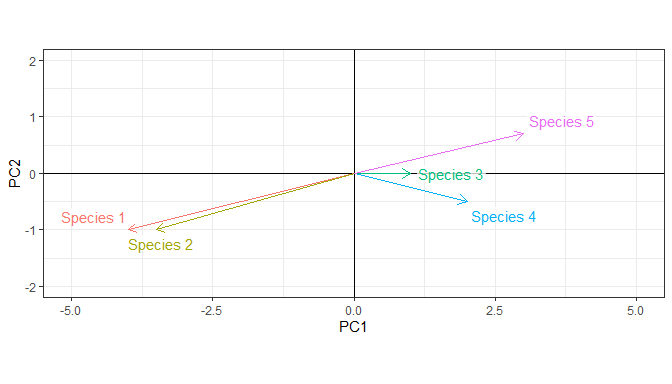The use of position_nudge_center() together with repulsion, shown above, results a much better plot than using only repulsion.

ggplot(df1, aes(x=PC1, y = PC2, label = Species, colour = Species)) +
geom_hline(aes(yintercept = 0), linewidth = .2) +
geom_vline(aes(xintercept = 0), linewidth = .2) +
geom_segment(aes(x = 0, y = 0, xend = PC1, yend = PC2),
arrow = arrow(length = unit(0.1, "inches"))) +
geom_label_repel(label.size = NA,
fill = rgb(red = 1, green = 1, blue = 1, alpha = 0.75)) +
xlim(-5, 5) +
ylim(-2, 2) +
# Stadard settings for displaying biplots
coord_fixed() +
theme(legend.position = "none")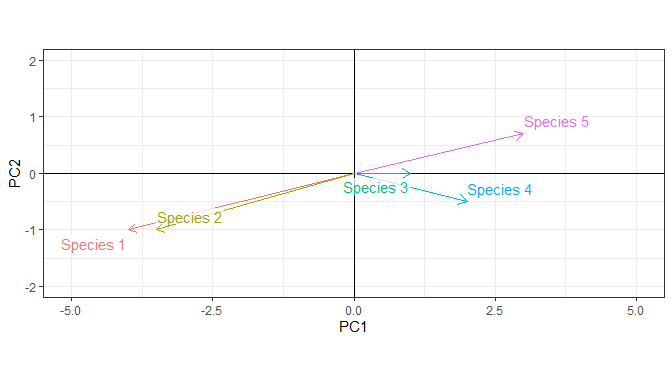The use of position_nudge_center() together with repulsion, shown above, results a much better plot than using only nudging. In this case, the default justification to the center needs to be overidden.

ggplot(df1, aes(x=PC1, y = PC2, label = Species, colour = Species)) +
geom_hline(aes(yintercept = 0), linewidth = .2) +
geom_vline(aes(xintercept = 0), linewidth = .2) +
geom_segment(aes(x = 0, y = 0, xend = PC1, yend = PC2),
arrow = arrow(length = unit(0.1, "inches"))) +
geom_label(position = position_nudge_center(x = 0.2, y = 0.01,
center_x = 0, center_y = 0),
label.size = 0,
vjust = "outward", hjust = "outward",
fill = rgb(red = 1, green = 1, blue = 1, alpha = 0.75)) +
xlim(-5, 5) +
ylim(-2, 2) +
# Stadard settings for displaying biplots
coord_fixed() +
theme(legend.position = "none")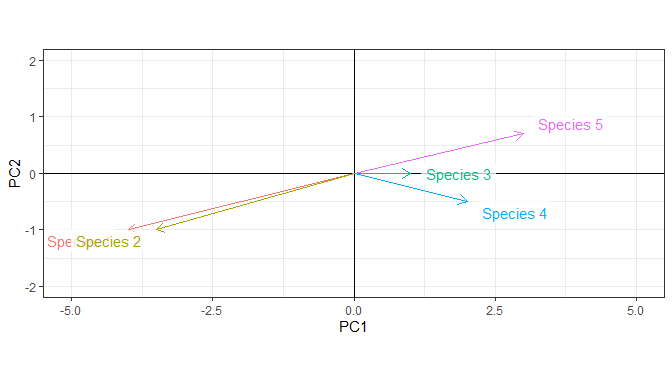Of course, nudging and justification could be manually adjusted for each label, but here we are concerned with approaches that avoid manual tweaking.

### Aligned data labels

Function position_nudge_to() nudges to a given position instead of using the same shift for each observation. It can be used to align labels for points that are not themselves aligned.

ggplot(df, aes(x, y, label = ifelse(x < 0.5, "", l) )) +
geom_point() +
geom_text_repel(position =
position_nudge_to(x = 2.3),
min.segment.length = 0,
segment.color = "red",
arrow = arrow(length = unit(0.015, "npc")),
direction = "y") +
expand_limits(x = 3)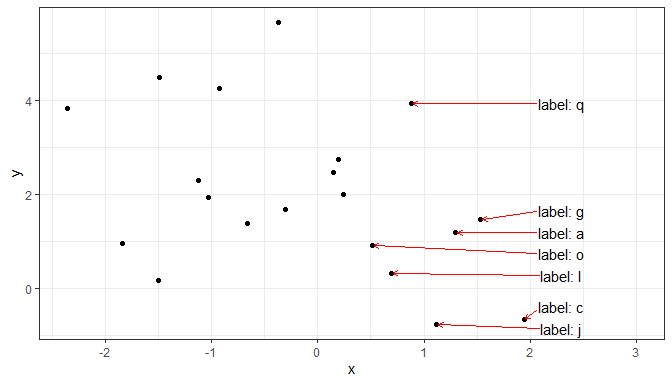By providing two values for nudging with opposite sign, we can add labels alternating between sides. We use here geom_text_s() but other geometries could have been used as well. How the data labels been closer together repulsion would have been needed in addition to nudging.

size_from_area <- function(x) {sqrt(max(0, x) / pi)}

df2 <- data.frame(b = exp(seq(2, 4, length.out = 10)))

ggplot(df2, aes(1, b, size = b)) +
geom_text_s(aes(label = round(b,2)),
position = position_nudge_to(x = c(1.1, 0.9)),
geom_point() +
scale_size_area() +
xlim(0, 2) +
theme(legend.position = "none")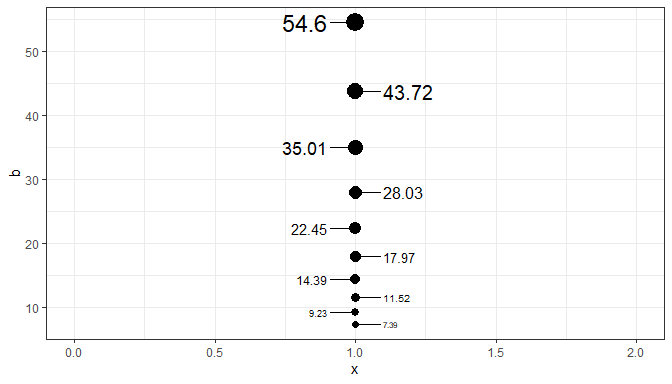It is also useful when labeling curves than end at different positions along the x axis. In this example we avoid overlaps with repulsion along the y axis. The data set used in this example is dynamic, so we use nudging to a position that is dynamicaly computed from the data.

keep <- c("Israel", "United States", "European Union", "China", "South Africa", "Qatar",
"Argentina", "Chile", "Brazil", "Ukraine", "Indonesia", "Bangladesh")

data$date <- ymd(data$date)

data %>%
filter(location %in% keep) %>%
select(location, date, total_vaccinations_per_hundred) %>%
arrange(location, date) %>%
filter(!is.na(total_vaccinations_per_hundred)) %>%
mutate(location = factor(location),
location = reorder(location, total_vaccinations_per_hundred)) %>%
group_by(location) %>% # max(date) depends on the location!
mutate(label = if_else(date == max(date),
as.character(location),
"")) -> owid

ggplot(owid,
aes(x = date,
y = total_vaccinations_per_hundred,
color = location)) +
geom_line() +
geom_text_repel(aes(label = label),
size = 3,
position = position_nudge_to(x = max(owid$date) + days(30)), segment.color = 'grey', point.size = 0, box.padding = 0.1, point.padding = 0.1, hjust = "left", direction = "y") + scale_x_date(expand = expansion(mult = c(0.05, 0.2))) + labs(title = "Cumulative COVID-19 vaccination doses administered per 100 people", y = "", x = "Date (year-month)") + theme_bw() + theme(legend.position = "none")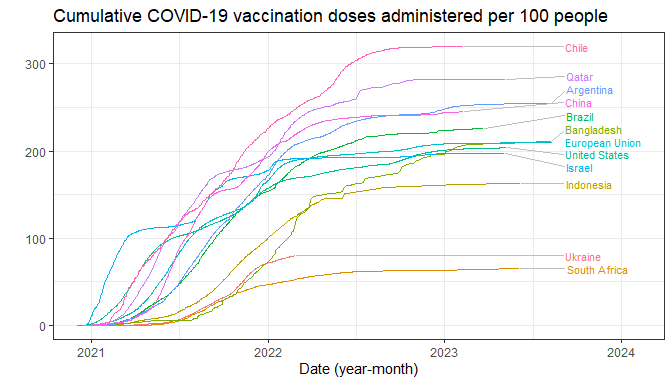In the call to position_nudge_to() we passed a vector of length one as argument for y, but both x and y also accept longer vectors. In other words, this position function makes it possible manual positioning of text and labels. In the next example we decrease the forces used for repulsion and the padding so that the labels remain close together. In this way, we can label the observations on the rug of a combined point and rug plot. ggplot(df, aes(x, y, label = round(x, 2))) + geom_point(size = 3) + geom_text_repel(position = position_nudge_to(y = -2.7), size = 3, angle = 90, hjust = 0, box.padding = 0.05, min.segment.length = Inf, direction = "x", force = 0.1, force_pull = 0.1) + geom_rug(sides = "b", length = unit(0.02, "npc"))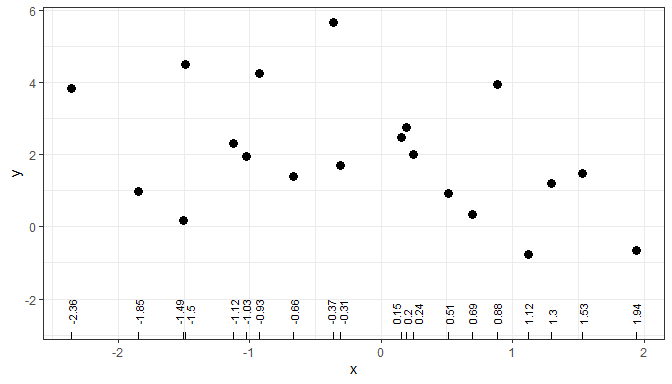### Clouds of observations In many cases data are distributed as a cloud with decreasing density towards edges. In some other cases, even with evely distributed observations, a certain partly systematic pattern of displacement of data labels is visually more attractive than a fully random one. In both cases, combining nudging and repulsion is usually an effective approach. We start with an examples showing a specific nudging pattern, and only later we combine them with repulsion. Function position_nudge_center() can nudge radially away from a focal point if both x and y are passed as arguments, or towards opposite sides of a vertical or horizontal virtual boundary line if only one of x or y is passed an argument. By default, the “center” is the centroid computed using mean(), but other functions or numeric values can be passed to override it. When data are sparse, such nudging may be effective in avoiding label overlaps, and in achieving a visually pleasing positioning. By default, split is away or towards the mean(). Here we allow repulsion to separate the labels (compare with the previous plot). ggplot(df, aes(x, y, label = l)) + geom_vline(xintercept = 0, linetype = "dashed") + geom_point() + geom_point_s(position = position_nudge_center(x = 0.3, center_x = 0), colour = "red")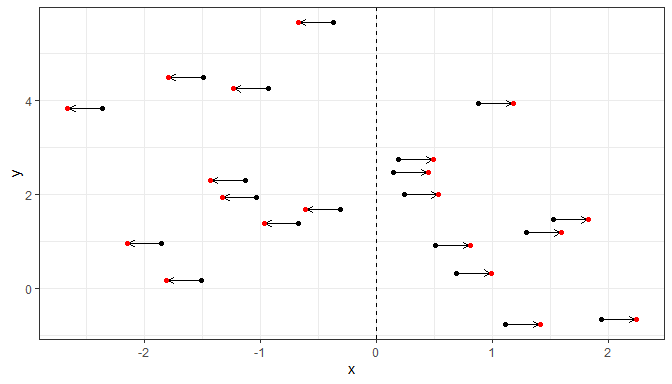In this second example we use repulsion and add data labels instead of displaced points. In all cases nudging shifts the coordinates giving a new x and/or y position that expands the limits of the corresponding scales to include the nudged coordinate values, but not necessarily the whole of justified text or labels. ggplot(df, aes(x, y, label = l)) + geom_vline(xintercept = 0, linetype = "dashed") + geom_point() + geom_text_repel(position = position_nudge_center(x = 0.3, center_x = 0), min.segment.length = 0)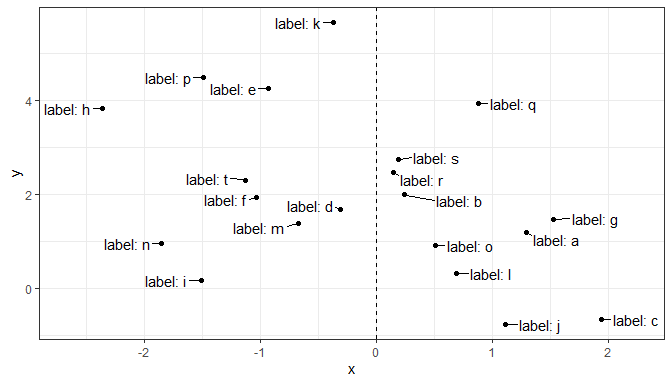We set a different split point as a constant value. ggplot(df, aes(x, y)) + geom_vline(xintercept = 1, linetype = "dashed") + geom_point() + geom_point_s(position = position_nudge_center(x = 0.3, center_x = 1), colour = "red")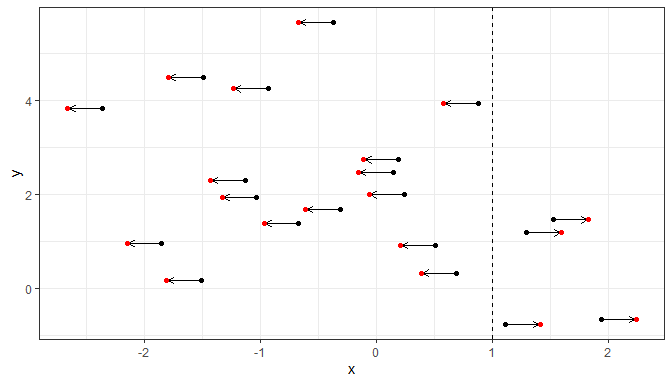We set a different split point as the value computed by a function function, by name. ggplot(df, aes(x, y)) + geom_vline(xintercept = median(df$x), linetype = "dashed") +
geom_point() +
geom_point_s(position =
position_nudge_center(x = 0.3, center_x = median),
colour = "red")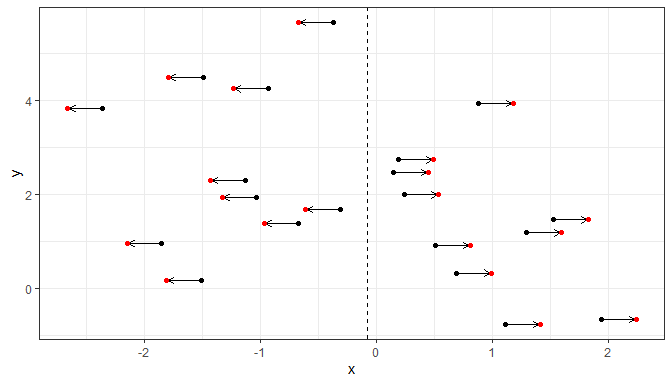We set a different split point as the value computed by an anonymous function. Here we split on the first quartile along x and y = 2.

ggplot(df, aes(x, y)) +
geom_point() +
geom_point_s(position =
position_nudge_center(x = 0.3, y = 0.3,
center_x = function(x) {
quantile(x,
probs = 1/4,
names = FALSE)
},
center_y = 2,
direction = "split"),
colour = "red")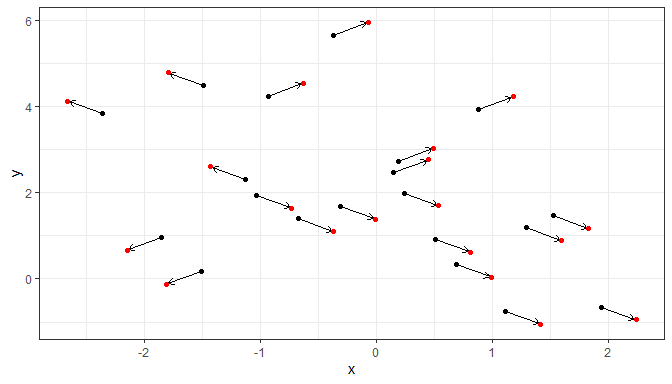The labels can be rotated as long as the geometry used supports this.

ggplot(df, aes(x, y, label = l)) +
geom_point() +
geom_text_repel(angle = 90,
position =
position_nudge_center(y = 0.1,
direction = "split"))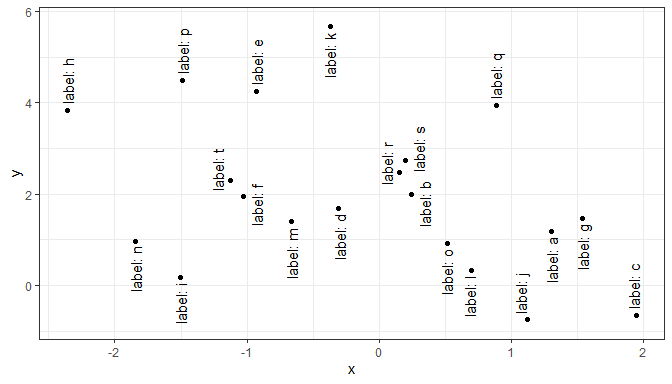By requesting nudging along x and y and setting direction = "split" nudging is applied according to the quadrants centred on the centroid of the data.

ggplot(df, aes(x, y)) +
stat_centroid(shape = "+", size = 5, colour = "red") +
geom_point() +
geom_point_s(position =
position_nudge_center(x = 0.2,
y = 0.3,
direction = "split"),
colour = "red")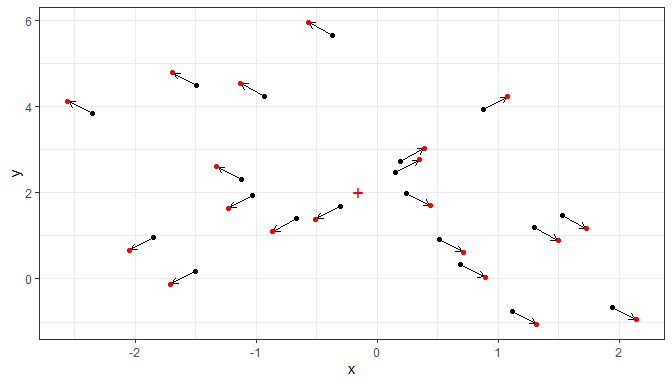ggplot(df, aes(x, y, label = l)) +
geom_point() +
geom_text_repel(position =
position_nudge_center(x = 0.1,
y = 0.15,
direction = "split"))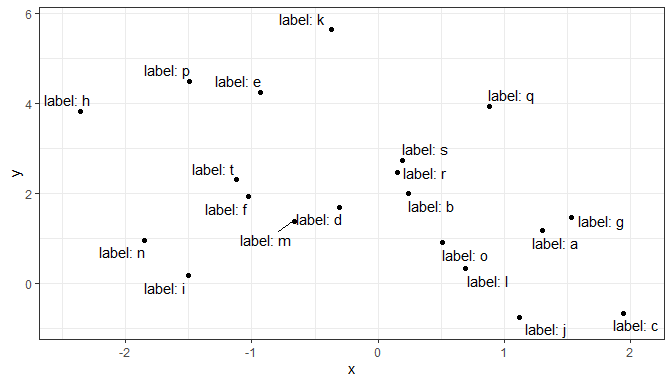With direction = "radial", the distance nudged away from the center is the same for all labels.

ggplot(df, aes(x, y)) +
stat_centroid(shape = "+", size = 5, colour = "red") +
geom_point() +
geom_point_s(position =
position_nudge_center(x = 0.25,
y = 0.4,
colour = "red")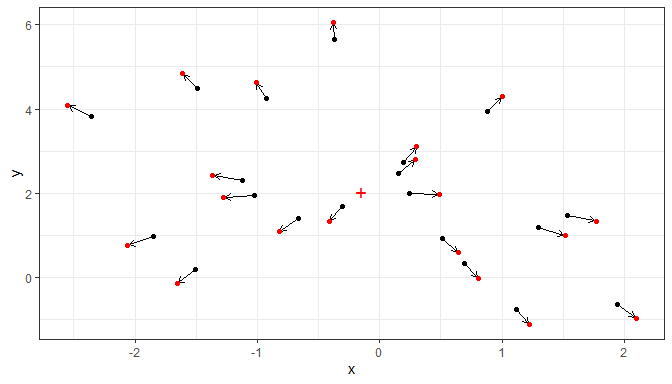ggplot(df, aes(x, y, label = l)) +
geom_point() +
geom_text_repel(position =
position_nudge_center(x = 0.25,
y = 0.4,
min.segment.length = 0)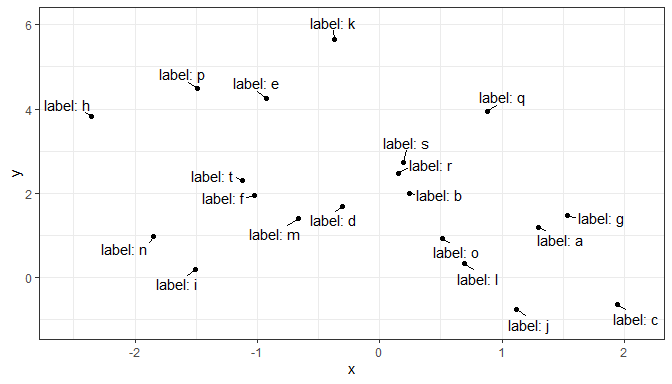As shown above for direction = "split" we can set the coordinates of the center also with direction = "radial".

We can also set the justification of the text labels although repulsion usually works best with labels justified at the centre, which is the default in geom_text_repel().

ggplot(df, aes(x, y, label = l)) +
geom_point() +
geom_text_repel(position =
position_nudge_center(x = 0.125,
y = 0.25,
center_x = 0,
center_y = 0,
min.segment.length = 0,
hjust = "outward", vjust = "outward") +
expand_limits(x = c(-2.7, +2.3))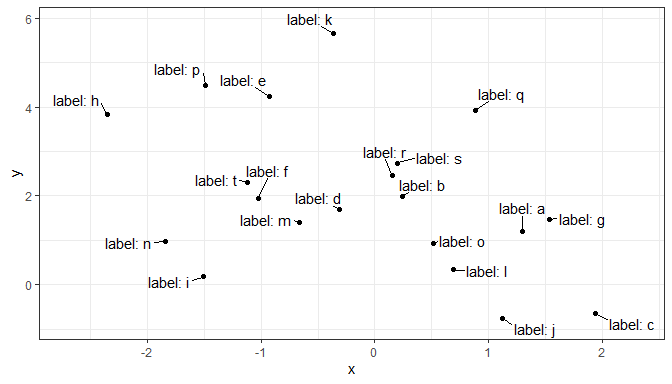Nudging along one axis, here x, and setting the repulsion direction along the other axis, here y, tends to give a pleasant arrangement of labels.

ggplot(df, aes(x, y, label = l)) +
geom_point() +
geom_text_repel(position =
position_nudge_center(x = 0.2,
center_x = 0,
direction = "split"),
aes(hjust = "outward"),
direction = "y",
min.segment.length = 0) +
expand_limits(x = c(-3, 3))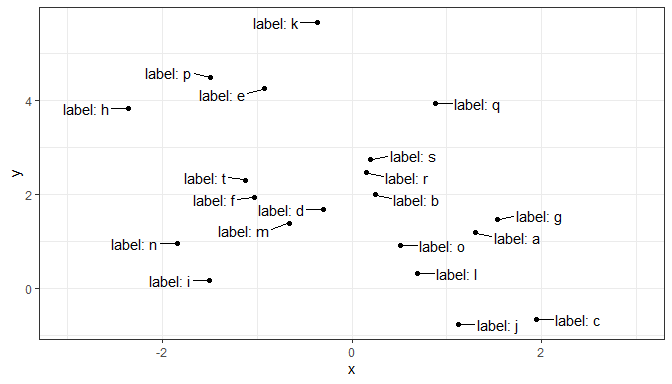When some regions have a high density of observations we may wish to only label those in the lower density regions. To automate this, we can use statistics stat_dens2d_labels() or stat_dens1d_labels() that replace the labels with "" but retain all rows in data so that repulsion away from all points is achieved. In contrast, stat_dens2d_filter() or stat_dens1d_filter() subset data using identical criteria.

ggplot(df, aes(x, y, label = l)) +
geom_point() +
stat_dens2d_labels(geom = "text_repel",
keep.fraction = 1/3,
position =
position_nudge_center(x = 0.2,
center_x = 0,
direction = "split"),
aes(hjust = ifelse(x < 0, 1, 0)),
direction = "y",
min.segment.length = 0) +
stat_dens2d_filter(geom = "point",
keep.fraction = 1/3,
shape = "circle open", size = 3) +
expand_limits(x = c(-3, 3))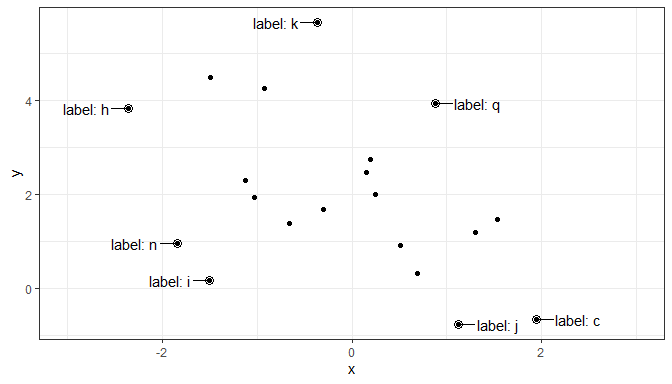We create a set of example data with a denser distribution.

random_string <- function(len = 3) {
paste(sample(letters, len, replace = TRUE), collapse = "")
}

# Make random data.
set.seed(1001)
d <- tibble::tibble(
x = rnorm(100),
y = rnorm(100),
group = rep(c("A", "B"), c(50, 50)),
lab = replicate(100, { random_string() })
)
ggplot(data = d, aes(x, y, label = lab, colour = group)) +
geom_point() +
stat_dens2d_labels(geom = "text_repel",
keep.fraction = 0.45)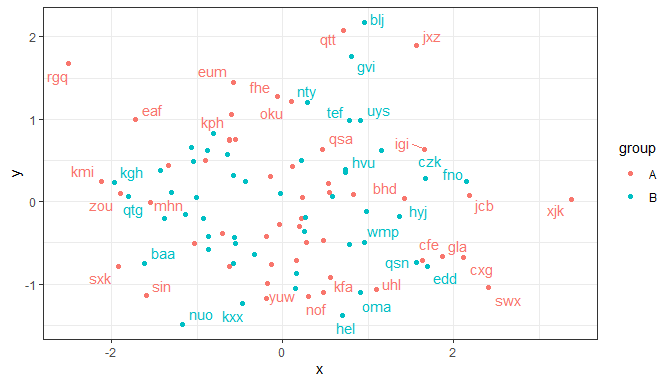With geom_label_repel one usually needs to use a smaller value for keep.fracton, or a smaller size, as labels use more space on the plot than the test alone.

Additional arguments can be used to change the angle and position of the text, but may give unexpected output when labels are long as the repulsion algorithm “sees” always a rectangular bounding box that is not rotated. With short labels or angles that are multiples of 90 degrees, there is no such problem. Please, see the documentation for ggrepel::geom_text_repel and ggrepel::geom_label_repel for the various ways in which both repulsion and formatting of the labels can be adjusted.

Using NA as argument to label.fill makes the observations with labels set to NA incomplete, and such rows in data are skipped when rendering the plot, before the repulsion algorithm is active. This can lead to overlap between text and points corresponding to unlabelled observations. Whether points are occluded depends on the order of layers and transparency, the occlusion can remain easily unnoticed with geom_label and geom_label_repel. We keep geom_point as the topmost layer to ensure that all observations are visible.

ggplot(data = d, aes(x, y, label = lab, colour = group)) +
stat_dens2d_labels(geom = "label_repel",
keep.fraction = 0.2,
label.fill = NA) +
geom_point()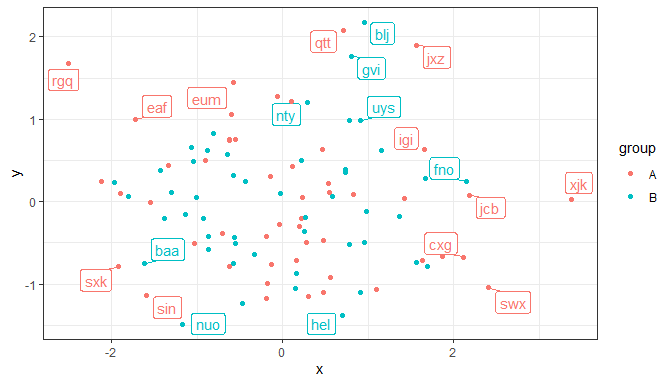The 1D versions work similarly but assess the density along only one of x or y. In other respects than orientation and the parameters passed internally to stats::density() the examples given earlier for stat_dens2d_labels() also apply stat_dens1d_labels().

An example for a plot based on an enhancement suggested in an issue raised at GitHub by Michael Schubert, made possible by parameter keep.these added for this and similar use cases.

library(ggplot2)
library(ggpp)
library(ggrepel)
syms = c(letters[1:5], LETTERS[1:5], 0:9)
labs = do.call(paste0, expand.grid(syms, syms))
dset = data.frame(x=rnorm(1e3), y=rnorm(1e3), label=sample(labs, 1e3, replace=TRUE))
ggplot(dset, aes(x=x, y=y, label = label)) +
geom_point(colour = "grey85") +
stat_dens2d_filter(geom = "text_repel",
position = position_nudge_centre(x = 0.1,
y = 0.1,
keep.number = 50,
keep.these = c("aA", "bB", "cC"),
min.segment.length = 0) +
theme_bw()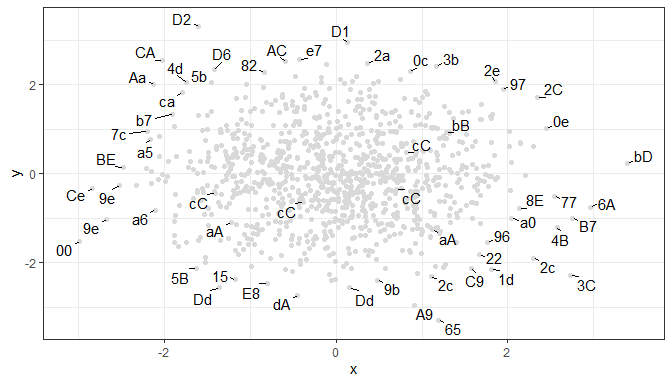### Lines, curves and observations along them

Function position_nudge_line() nudges away from a line, which can be a user supplied straight line as well as a smooth spline or a polynomial fitted to the observations themselves. The nudging is away and perpendicular to the local slope of the straight or curved line. It relies on the same assumptions as linear regression, assuming that x values are not subject to error. This in most cases prevents labels from overlapping a curve fitted to the data, even if not exactly based on the same model fit. When observations are sparse, this may be enough to obtain a nice arrangement of data labels, otherwise, it can be used in combination with repulsive geometries.

set.seed(16532)
df <- data.frame(
x = -10:10,
y = (-10:10)^2,
yy = (-10:10)^2 + rnorm(21, 0, 4),
yyy = (-10:10) + rnorm(21, 0, 4),
l = letters[1:21]
)

The first, simple example shows that position_nudge_line() has shifted the direction of the nudging based on the alignment of the observations along a line. One could, of course, have in this case passed suitable values as arguments to x and y using position_nudge() from package ‘ggplot2’. However, position_nudge_line() will work without change with curves or with observations not exactly falling on a line.

In the plots that follow the original positions are shown in black and the nudged ones in red, with an arrow showing the displacement introduced by nudging.

ggplot(df, aes(x, 2 * x, label = l)) +
geom_point() +
geom_abline(intercept = 0, slope = 2, linetype = "dotted") +
geom_point_s(position = position_nudge_line(x = -1, y = -2),
colour = "red")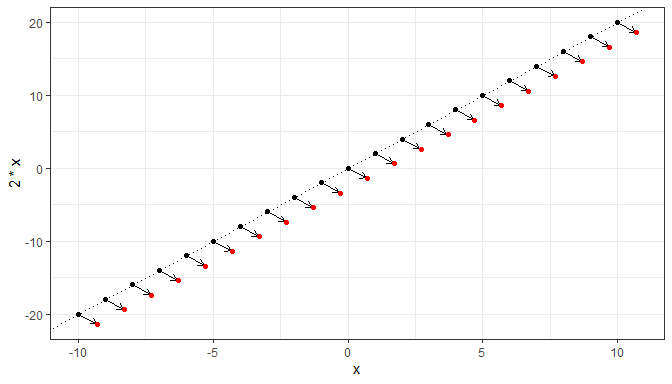With observations with variation in y, a linear model fit may need to be used. In this case fitted twice, once in stat_smooth() and once in position_nudge_line().

ggplot(subset(df, x >= 0), aes(x, yyy)) +
geom_point() +
stat_smooth(method = "lm", formula = y ~ x) +
geom_point_s(position = position_nudge_line(x = 0, y = 1.2,
method = "lm",
direction = "split"),
colour = "red")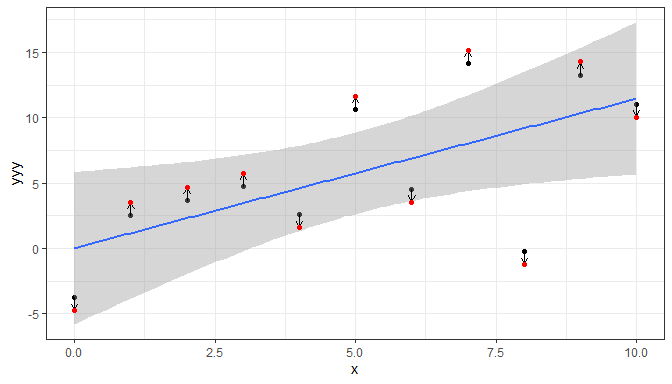With lower variation in y, we can pass to line_nudge a multiplier to keep labels outside of the confidence band.

ggplot(subset(df, x >= 0), aes(y, yy)) +
geom_point() +
stat_smooth(method = "lm", formula = y ~ x) +
geom_point_s(position = position_nudge_line(method = "lm",
x = 1.5, y = 3,
line_nudge = 2.75,
direction = "split"),
colour = "red")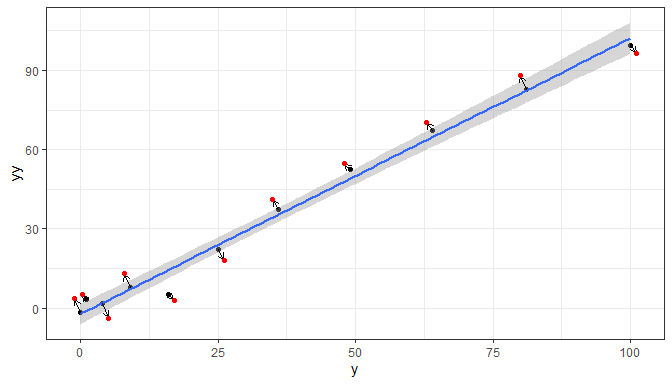If we want the nudging based on an arbitrary straight line not computed from data, we can pass the intercept and slope in a numeric vector of length two as an argument to parameter abline.

ggplot(subset(df, x >= 0), aes(y, yy)) +
geom_point() +
geom_abline(intercept = 0, slope = 1, linetype = "dotted") +
geom_point_s(position = position_nudge_line(abline = c(0, 1),
x = 3, y = 6,
direction = "split"),
colour = "red")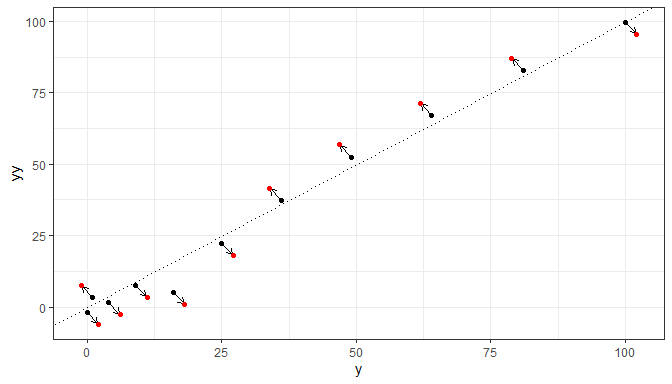More frequently observations follow curves rather than straight lines. If observations follow exactly a simple curve nudging away from the curve with position_nudge_line() can be very effective. In this case, the interpretation of values passed as arguments to parameters x and y of the position function differs from that in position_nudge(): positive values correspond to above and inside the curve and negative ones, the opposite direction.

The next plot shows the effect of nudging with the original positions as black dots and the nudged positions as red dots.

ggplot(df, aes(x, y)) +
geom_point() +
geom_line(linetype = "dotted") +
geom_point_s(position = position_nudge_line(x = 0.6, y = 6),
colour = "red")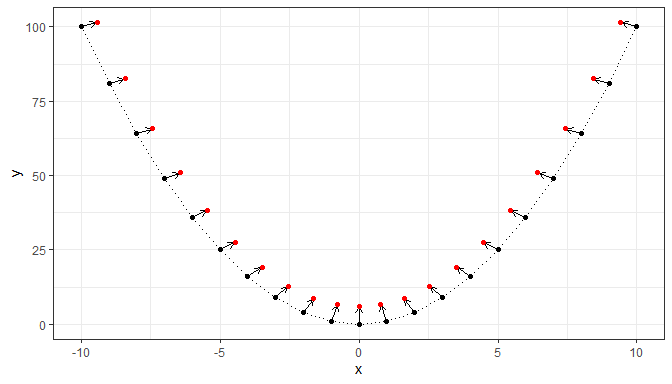Negative values passed as arguments to x and y correspond to labels below and outside the curve.

ggplot(df, aes(x, y)) +
geom_point() +
geom_line(linetype = "dotted") +
geom_point_s(position = position_nudge_line(x = -0.6, y = -6),
colour = "red")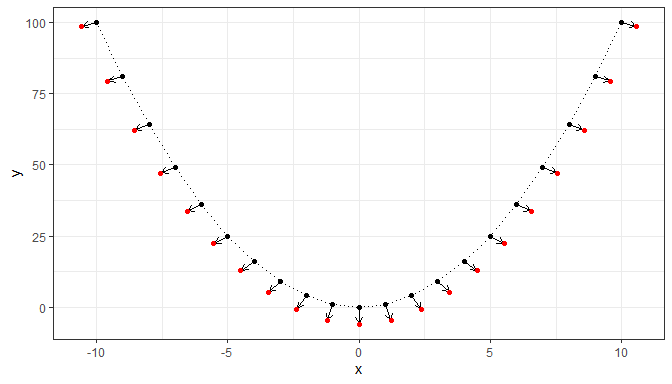When the observations include random variation along y, it is important that the smoother used for the line added to a plot and that passed to position_nudge_line() are similar. By default stat_smooth() uses "loess" and position_nudge_line() with method "spline", smooth.sline(), which are a good enough match.

ggplot(df, aes(x, yy)) +
geom_point() +
stat_smooth(method = "loess", formula = y ~ x) +
geom_point_s(position = position_nudge_line(x = 0.6, y = 6,
direction = "split"),
colour = "red")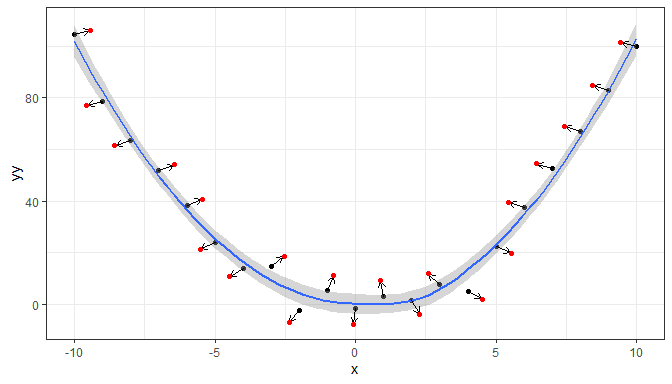We can use other geometries, or rather we need to use a repulsive geometry when the label text is long or the labels are crowded near the line. Combining repulsion and computed nudging is effective.

ggplot(df, aes(x, yy)) +
geom_point() +
stat_smooth(method = "loess", formula = y ~ x) +
geom_label_repel(aes(y = yy, label = paste("point", l)),
position = position_nudge_line(x = 0.6,
y = 8,
direction = "split"),
min.segment.length = 0)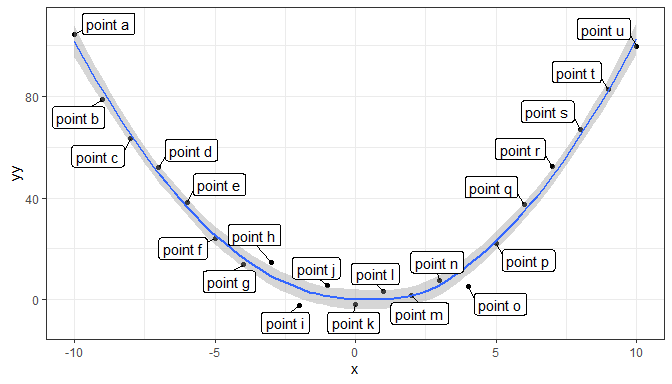We can see by comparing the plot above with that below, that combining nudging away from a line with repulsion results in a more pleasant positioning of the data labels.

ggplot(df, aes(x, yy)) +
geom_point() +
stat_smooth(method = "loess", formula = y ~ x) +
geom_label_repel(aes(y = yy, label = paste("point", l)),
min.segment.length = 0)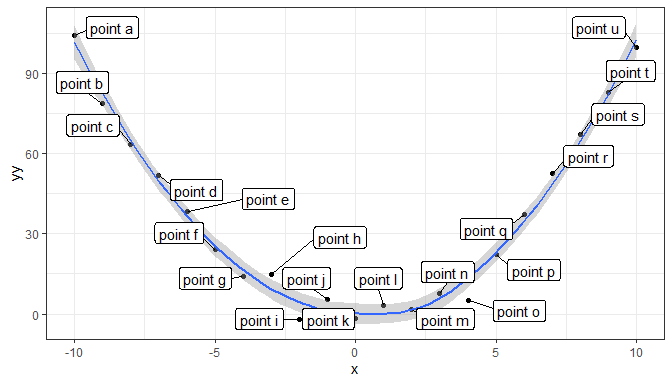Nudging alone, as shown next, results in overlaps and clipping.

ggplot(df, aes(x, yy)) +
geom_point() +
stat_smooth(method = "loess", formula = y ~ x) +
geom_label_s(aes(y = yy, label = paste("point", l)),
position = position_nudge_line(x = 0.6,
y = 8,
direction = "split"),
min.segment.length = 0)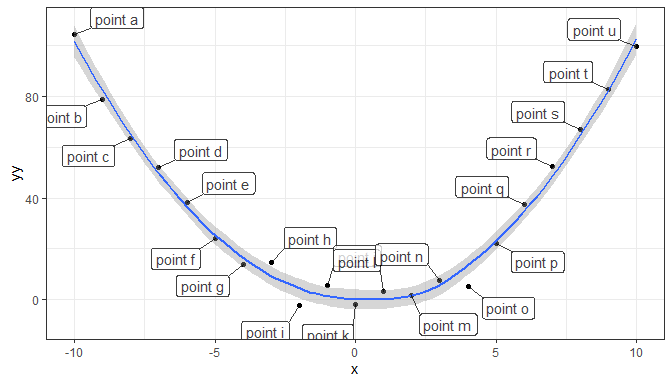While box.padding in geom_text_repel() controls the separation among data labels as well as between data labels and points, in geom_label_s() it controls only the distance between the end of the segment and the data label.

When fitting a polynomial, "lm" should be the argument passed to method and a model formula preferably based on poly(), setting raw = TRUE, as argument to formula.

Currently no other methods are implemented in position_nudge_line().

In the case of data labels that are small, a single character in the next example, we also benefit from nudging if they are near a fitted line. Nudging plus repulsion, shown next, will be compared to alternatives. In this case we assume no linking segments are desired as there is enough space for the data labels to remain near the observations.

ggplot(df, aes(x, yy)) +
geom_point() +
stat_smooth(method = "lm",
formula = y ~ poly(x, 2, raw = TRUE)) +
geom_text_repel(aes(y = yy, label = l),
position = position_nudge_line(method = "lm",
formula = y ~ poly(x, 2, raw = TRUE),
x = 0.5,
y = 5,
direction = "split"),
min.segment.length = Inf)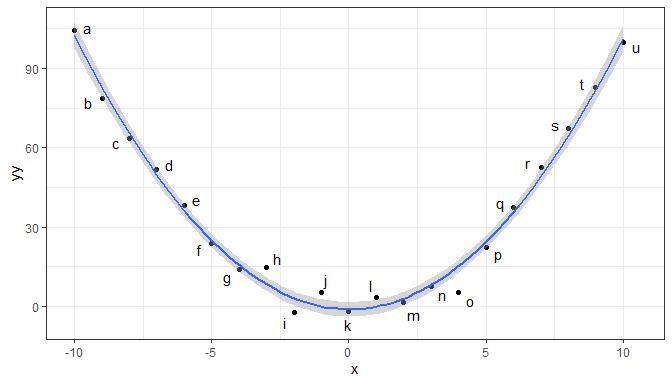Using nudging alone there is little difference, but there is always the posibility of overlaps, so using nudging plus repulsion as above is safer.

ggplot(df, aes(x, yy)) +
geom_point() +
stat_smooth(method = "lm",
formula = y ~ poly(x, 2, raw = TRUE)) +
geom_text(aes(y = yy, label = l),
position = position_nudge_line(method = "lm",
formula = y ~ poly(x, 2, raw = TRUE),
x = 0.5,
y = 5,
direction = "split"))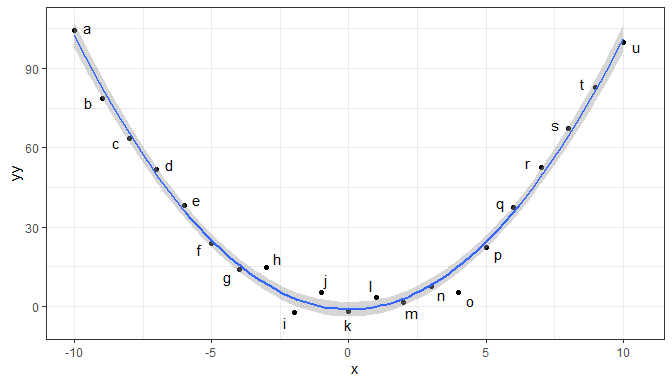Repulsion without nudging as shown below is unsatisfactory in this case, i.e., adding nudging displaces the data labels in the desired direction. Compare the plot below using no nudging with the one above.

ggplot(df, aes(x, yy)) +
geom_point() +
stat_smooth(method = "lm",
formula = y ~ poly(x, 2, raw = TRUE)) +
geom_text_repel(aes(y = yy, label = l),
min.segment.length = Inf)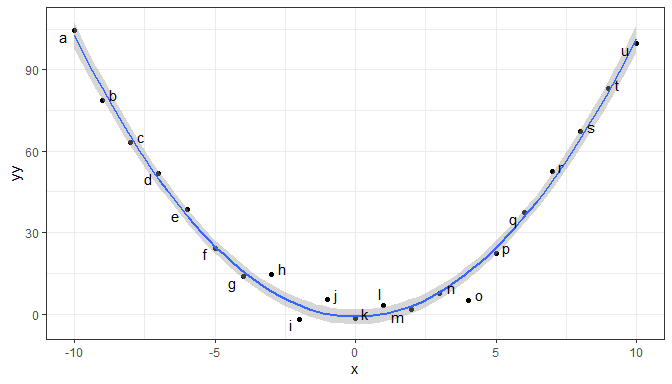## Combined position functions

Using position_stacknudge() together geom_label_repel() makes it possible to use repulsion for labeling sections of stacked column plots.

df <- tibble::tribble(
~y, ~x, ~grp,
"a", 1,  "some long name",
"a", 2,  "other name",
"b", 1,  "some name",
"b", 3,  "another name",
"b", -1, "some long name"
)

ggplot(data = df, aes(x, y, group = grp)) +
geom_col(aes(fill = grp), width=0.5) +
geom_vline(xintercept = 0) +
geom_label_repel(aes(label = grp),
position = position_stacknudge(vjust = 0.5, y = 0.4),
label.size = NA)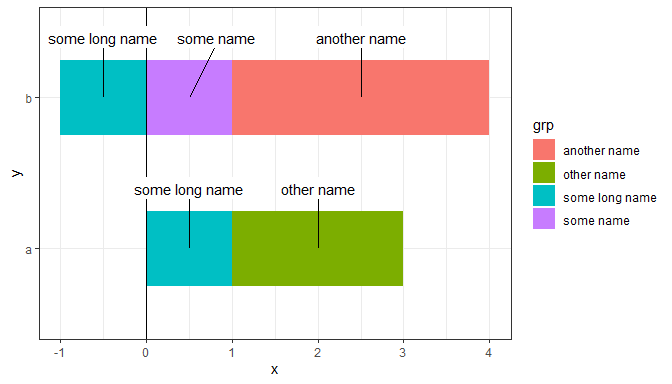## Acknowledgements

I warmly thank Kamil Slowikowski for agreeing to make changes in ‘ggrepel’ that make the use of ‘ggrepel’ together with ‘ggpp’ possible and smooth. This document shows some use examples, but surely new ones will be found by users of R and ‘ggplot2’.